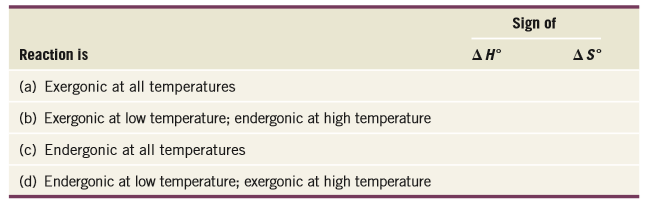# Problem: Complete the following table by adding + and - signs to the ΔH° and ΔS° columns so as to correspond to the effect of temperature on a reversible reaction.

###### Problem Details

Complete the following table by adding + and - signs to the  ΔH° and  ΔS° columns so as to correspond to the effect of temperature on a reversible reaction.What scientific concept do you need to know in order to solve this problem?

Our tutors have indicated that to solve this problem you will need to apply the Gibbs Free Energy concept. You can view video lessons to learn Gibbs Free Energy. Or if you need more Gibbs Free Energy practice, you can also practice Gibbs Free Energy practice problems.

What is the difficulty of this problem?

Our tutors rated the difficulty ofComplete the following table by adding + and - signs to the ...as high difficulty.

How long does this problem take to solve?

Our expert Organic tutor, Chris took 6 minutes and 21 seconds to solve this problem. You can follow their steps in the video explanation above.

What textbook is this problem found in?

Our data indicates that this problem or a close variation was asked in Organic Chemistry - Carey 8th Edition. You can also practice Organic Chemistry - Carey 8th Edition practice problems.